# Money Exchange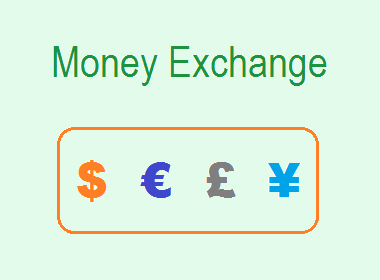In the world, each country has its own currency. For example, the Currencies of Thailand, UK, and Nepal are Bhat, Pound Sterling, and Rupees respectively. The values of the currencies of different countries are different. The exchange of a currency of a particular country with the currency of another country is called Money Exchange.

There are different rates for the selling and buying of foreign currencies. The bank or money exchanger makes some profit from the difference in selling and buying rates.

In this article, we will study about the exchange of Nepali currency with the currency of other countries.

## Chain Rule

The Chain Rule is one of the popular methods by which we can calculate or convert currencies. The method of the Chain Rule is as follows:

If A = B, B = C and C = x (say)

Then,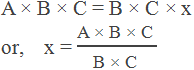### Worked Out Examples

Example 1: If Indian Currency IRs. 100 = Nepali Currency NRs. 160, convert NRs. 3200 to Indian Currency IRs. by using the chain rule.

Solution:

Let,   IRs. x = NRs. 3200

NRs. 160 = IRs. 100

By using the chain rule,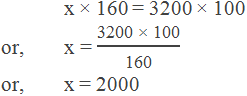We can exchange NRs. 3200 for Rs. 2000 Indian Currency.

Example 2: If 25 US dollars can be exchanged for IRs.1562.50. The exchange rate of IRs. 100 is equal to NRs. 160. Find how many US dollars can be exchanged for NRs. 1500.

Solution:

Let,   \$ x = NRs. 1500

IRs. 1562.50 = \$ 25

NRs. 160 = IRs. 100

By using the chain rule,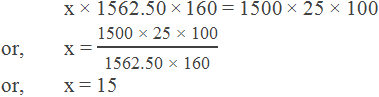15 US dollars can be exchanged for NRs. 1500.

Example 3: If the exchange rate of \$ 100 is equal to £ 68.90. \$ 125 can be exchanged with NRs. 13125. Find how many NRs. are needed to exchange for £ 75.

Solution:

Let,   NRs. x = £ 75

£ 68.90 = \$ 100

\$ 125 = NRs. 13125

By using the chain rule,NRs. 11429.60 can be exchanged for £ 75.

Example 4: NRs. 875 can exchange 1000 Japanese Yen. 1 Saudi Riyal equals to NRs. 27.50. Find How many Japanese Yen should be exchanged with 50 Saudi Riyal.

Solution:

Let,   x Japanese Yen = 50 Saudi Riyal

1 Saudi Riyal = NRs. 27.50

NRs. 875 = 1000 Japanese Yen

By using the chain rule,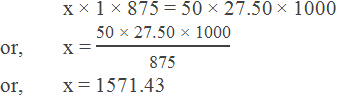1571.43 Japanese Yen can be exchanged with 50 Saudi Riyal.

Example 5: If a money exchanger charges a 0.5% commission, find Nepali rupees NRs. required to exchange 3200 Euro if 1 Euro = NRs. 140.44.

Solution: Here,

1 Euro = NRs. 140.44

3200 Euro = NRs. 140.44 × 3200 = NRs. 449408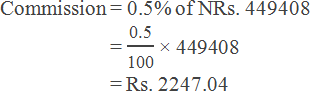Total NRs required to exchange for 3200 Euro

= Rs. 449408 + 2247.04

= NRs. 451655.04

Example 6: According to the money exchange rate by the National Bank, the buying and the selling rates of 1 US dollar are NRs. 103.72 and NRs. 104.32 respectively, then

i. How many US dollars can be exchanged with NRs. 57376?

ii. How many NRs. can be exchanged with US dollar 500?

Solution: Here,

Selling rate = NRs. 104.32

i. Let, \$ x can be exchanged with NRs. 57376

\$ x = NRs. 57376

NRs. 104.32 = \$ 1

By using chain rule,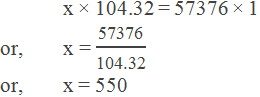\$ 550 can be exchanged with NRs. 57376.

ii. Let, NRs. x can be exchanged for \$ 500

NRs. x = \$ 500

\$ 1 = NRs. 103.72

By using the chain rule,

x × 1 = 500 × 103.72

or,     x = 51860

NRs. 51860 can be exchanged for Rs. 500.

Example 7: Surekha bought US dollars for NRs. 55424 at the rate of 1 dollar = Rs. 108.25. After some days, Nepali currency is devaluated by 10% with comparison to US dollars. She sold the dollars after devaluation. How much profit or loss does she got?

Solution: Here,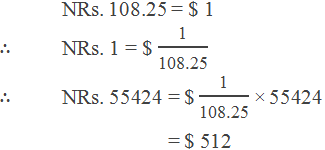She purchased \$ 512 before the devaluation.

After devaluation by 10%,

\$ 1 = NRs. 108.25 + 10% of 108.25

= NRs. 108.25 + 10.825

= NRs. 119.075

Now,

\$ 512 = NRs. 119.075 × 512 = Rs. 60966.40

Profit = NRs. 60966.40 – 55424

= NRs. 5542.40

If you have any questions or problems regarding the Money Exchange, you can ask here, in the comment section below.

1.If the value of nepali currency is devaluation then shouldn't there be 108.25 – 10% of 108.25? Please explain

1.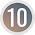If the value of nepali currency is devaluated in comparison to the foreign currency then you will get more value in nepali currency for a unit foreign currency than you could get before the devaluation. Because, value of nepali currency has been decreased and there would have more nepali money for a unit foreign currency. So we should add there 10% i.e. 108.25 + 10% of 108.25.

2.How many US S can be bought with the amount of Nepali currency that can buy £200 after allowing 10% devaluation in Nepali currency and 4% bank commission? (Before devaluation selling rate, 1 US S- Rs. 109.45, buying rate £ 1 = Rs 150 26)

1.Solution:

Here,
1 US \$ = Rs. 109.45
£ 1 = Rs. 150.26

After devaluation,
1 US \$ = Rs. 109.45 + 10% of 109.45 = 120.395
£ 1 = Rs. 150.26 + 10% of 150.26 = 165.286

∴ £ 200 = Rs. 165.286 × 200 – 4% commission = Rs. 31734.912
∴ Rs. 31734.912 = 31734.912/120.395 = 263.59 US \$

∴ 263.59 US \$ can be bought.